Last updated on 2/11/20

## Play with variables

You know how to use JavaScript to display values. However, for a program to be truly useful, it must be able to store data, like information entered by a user. Let's check that out.

### Variables

#### Role of a variable

A computer program stores data using variables. A variable is an information storage area. We can imagine it as a box in which you can put and store things!

#### Variable properties

A variable has three properties:

• Its name, which identifies it. A variable name may contain upper and lower case letters, numbers (not in the first position, ex.`1stvariable` ) and characters like the dollar (\$) or underscore (_).

• Its value, which is the data stored in the variable.

• Its type, which determines the role and actions available to the variable.

#### Declaring a variable

Before you can store information in a variable, you have to create it! This is called declaring a variable. Declaring a variable means the computer reserves memory in which it will store the variable. The program can then read or write data in this memory area by manipulating the variable.

Here's a code example that declares a variable and shows its contents:

``````var a;
console.log(a);``````

In JavaScript, you declare a variable with the `var` keyword followed by the variable name. In this example, the variable created is called `a`.

Add the above code to your `chapter-2`  JavaScript file, and open it in Chrome. You'll see the following: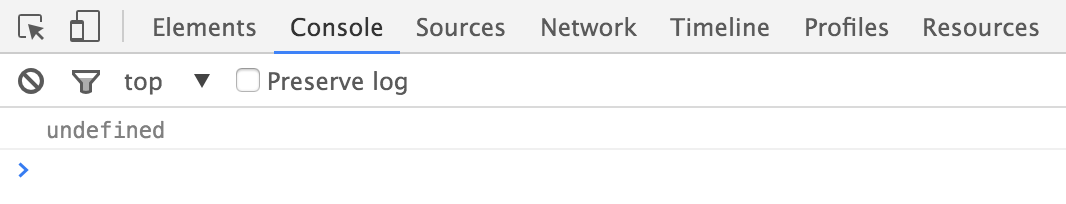Note that the result is `undefined` ! This is a JavaScript-type indicating no value. I declared the variable, calling it `a` but didn't give it a value!

### Assign values to variables

While a program is running, the value stored in a variable can change. To give new value to a variable, use the `=` operator, called an assignment operator.

Check out the example below:

``````var a;
a = 3.14;
console.log(a);``````

Here's what you'd get as a result.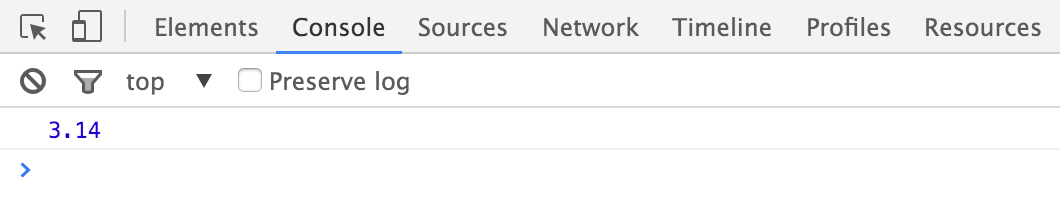We modified the variable by assigning it a value. `a = 3.14` means  `a` received the value `3.14`.

You can also combine declaring a variable and assigning it a value in one line. Just know that, within this line 1, you're doing two different things at once:

``````var a = 3.14;
console.log(a);``````

#### Increment a number variable

You can also increase or decrease a value of a number with `+=` and `++`. The latter is called an increment operator, as it allows incrementation (increase by 1) of a variable's value.

In the following example, lines 2 and 3 each increase the value of variable `b` by 1.

``````var b = 0; // b contains 0
b += 1; // b contains 1
b++; // b contains 2
console.log(b); // show 2``````

### Type conversion

An expression's evaluation can result in type conversion. These are called implicit conversions, as they happen automatically without the programmer's intervention. For example, using the `+` operator between a string and a number causes the concatenation of two values into a result: a string.

``````var f = 100;
console.log("The variable f contains the value: " + f);``````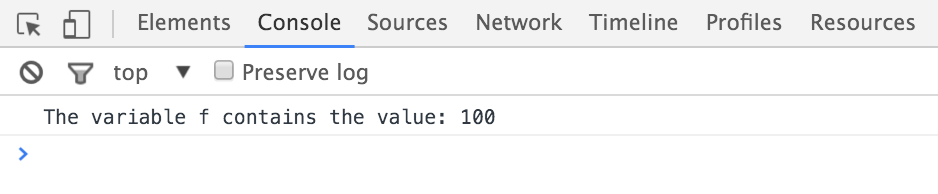JavaScript is very tolerant in terms of type conversion. However, sometimes conversion isn't possible. If a number fails to convert, you'll get the result `NaN` (Not a Number).

``````var g = "five" * 2;
console.log(g); // Shows NaN``````

Sometimes you'll wish to convert the value of another type. This is called explicit conversion. JavaScript has the `Number()` and `String()` commands that convert to numbers and strings.

``````var h = "5";
console.log(h + 1); // Concatenation: shows the string "51"
h = Number("5");
console.log(h + 1); // Addition: show the number 6``````

### User interactions

#### Entering and displaying information

Once you start using variables, you can write programs that exchange information with the user.

In the `chapter-2` /`js`  directory, create a file named `hello.js`  with the contents below.

``````var name = prompt ("Enter your first name:");

In the `chapter-2`  /`html ` directory, create a `hello.html` file and point it toward `hello.js` . Open the page, and an alert will pop up asking for your name.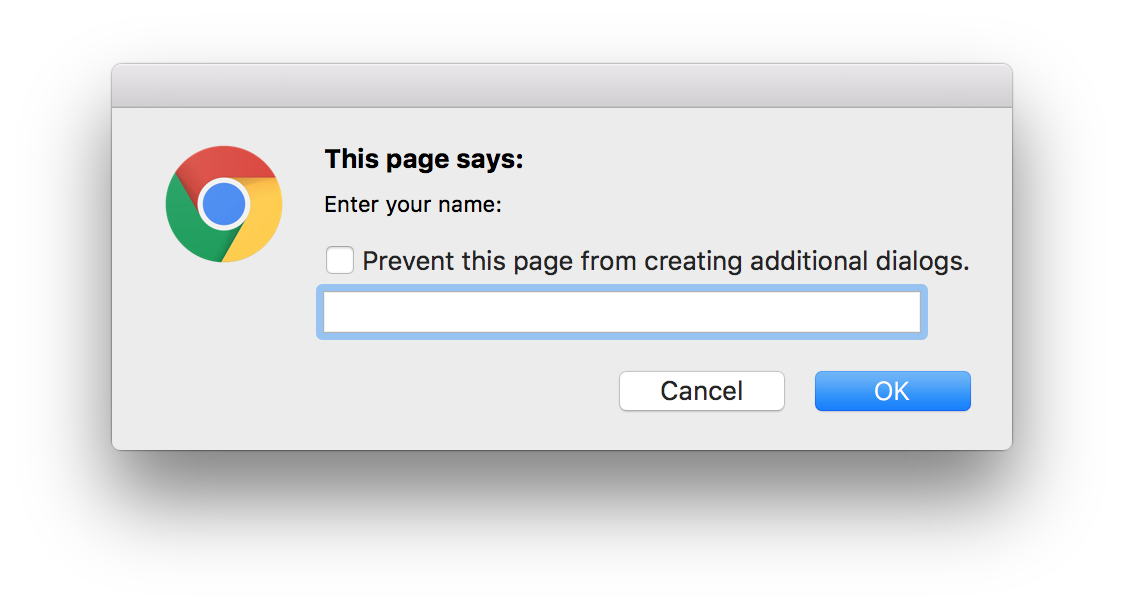This is the result of the JavaScript command `prompt("Enter your first name:")`

Type your name and click OK. You'll then get a personalized greeting.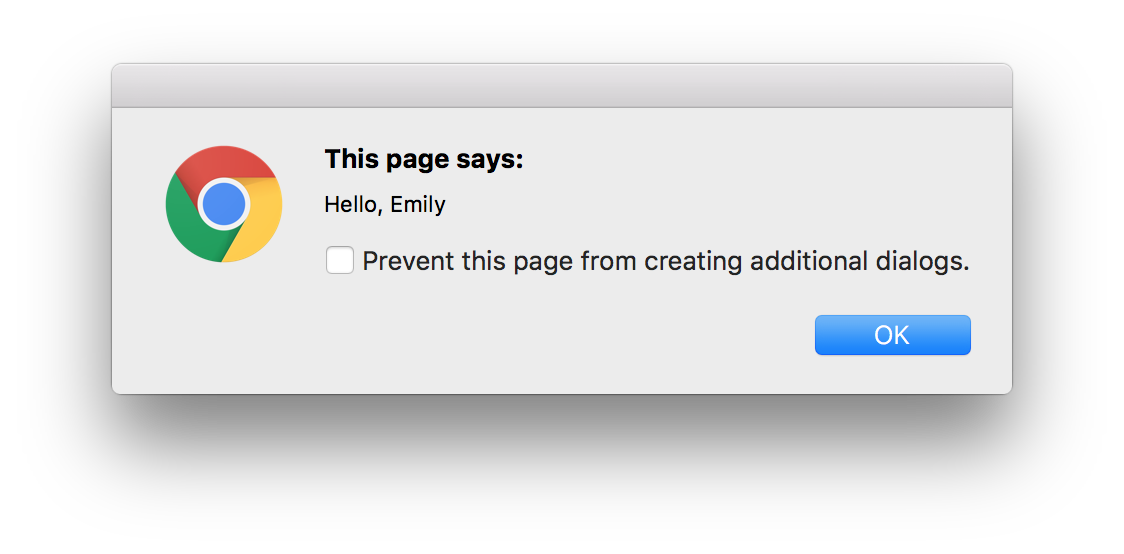The value you entered in the first dialog box has been stored as a string in the variable `name`.  The JavaScript command `alert()` then triggered the display of the second box, containing the result of the concatenation of the string `"Hello, "` with the value of the `name` variable.

### Naming variables

To close this chapter, let's discuss variable naming conventions. The computer doesn't care about variable names. You could name your variables using the classic example of a single letter (a, b, c...) or choose absurd names like `burrito` or `puppieskittens90210`.

Nonetheless, naming variables clearly can make your code much easier to read. Check out these two examples:

``````var nb1 = 5.5;
var nb2 = 3.14;
var nb3 = 2 * nb2 * nb1;
console.log(nb3);``````
``````var radius = 5.5;
var pi = 3.14;
var circumference = 2 * pi * radius;
console.log(circumference);``````

They function in the same way, but the second version is much easier to understand. What are some good ways to name variables?

• Choose meaningful names
The most important rule is to give each variable a name that reflects its role. This is the case in the second example above, where you use the word "radius" to actually indicate...well, a radius.

• Don't use reserved words
The keywords of JavaScript are reserved names. They should not be used as variable names. Here's the list of reserved words in JavaScript.